## Free Statistics

of Irreproducible Research!

Author's title
Author*Unverified author*
R Software Modulerwasp_meanplot.wasp
Title produced by softwareMean Plot
Date of computationSun, 22 Dec 2013 11:12:13 -0500
Cite this page as followsStatistical Computations at FreeStatistics.org, Office for Research Development and Education, URL https://freestatistics.org/blog/index.php?v=date/2013/Dec/22/t1387728756yr56zmc9ypwctim.htm/, Retrieved Sun, 05 Dec 2021 18:47:41 +0000
Statistical Computations at FreeStatistics.org, Office for Research Development and Education, URL https://freestatistics.org/blog/index.php?pk=232541, Retrieved Sun, 05 Dec 2021 18:47:41 +0000
QR Codes:Original text written by user:
IsPrivate?No (this computation is public)
User-defined keywords
Estimated Impact93
Family? (F = Feedback message, R = changed R code, M = changed R Module, P = changed Parameters, D = changed Data)
-     [Notched Boxplots] [werkloosheidscijf...] [2013-10-08 18:26:09] [6a1a05b03d1c87a66b915fc3d5866cc8]
- RMPD  [Harrell-Davis Quantiles] [consumtieprijs va...] [2013-12-21 15:50:38] [6a1a05b03d1c87a66b915fc3d5866cc8]
-   PD    [Harrell-Davis Quantiles] [] [2013-12-21 16:11:56] [6a1a05b03d1c87a66b915fc3d5866cc8]
- RMPD      [(Partial) Autocorrelation Function] [] [2013-12-21 18:23:11] [6a1a05b03d1c87a66b915fc3d5866cc8]
- RMPD          [Mean Plot] [] [2013-12-22 16:12:13] [4a7f7842fc88d649abcd00dd10ef7b6c] [Current]
Feedback Forum

Post a new message
Dataseries X:
125
123
117
114
111
112
144
150
149
134
123
116
117
111
105
102
95
93
124
130
124
115
106
105
105
101
95
93
84
87
116
120
117
109
105
107
109
109
108
107
99
103
131
137
135
124
118
121
121
118
113
107
100
102
130
136
133
120
112
109
110
106
102
98
92
92
120
127
124
114
108
106
111
110
104
100
96
98
122
134
133
125
118
116


 Summary of computational transaction Raw Input view raw input (R code) Raw Output view raw output of R engine Computing time 6 seconds R Server 'Gertrude Mary Cox' @ cox.wessa.net

\begin{tabular}{lllllllll}
\hline
Summary of computational transaction \tabularnewline
Raw Input & view raw input (R code)  \tabularnewline
Raw Output & view raw output of R engine  \tabularnewline
Computing time & 6 seconds \tabularnewline
R Server & 'Gertrude Mary Cox' @ cox.wessa.net \tabularnewline
\hline
\end{tabular}
%Source: https://freestatistics.org/blog/index.php?pk=232541&T=0

[TABLE]
[ROW][C]Summary of computational transaction[/C][/ROW]
[ROW][C]Raw Input[/C][C]view raw input (R code) [/C][/ROW]
[ROW][C]Raw Output[/C][C]view raw output of R engine [/C][/ROW]
[ROW][C]Computing time[/C][C]6 seconds[/C][/ROW]
[ROW][C]R Server[/C][C]'Gertrude Mary Cox' @ cox.wessa.net[/C][/ROW]
[/TABLE]
Source: https://freestatistics.org/blog/index.php?pk=232541&T=0

Globally Unique Identifier (entire table): ba.freestatistics.org/blog/index.php?pk=232541&T=0

As an alternative you can also use a QR Code:

The GUIDs for individual cells are displayed in the table below:

 Summary of computational transaction Raw Input view raw input (R code) Raw Output view raw output of R engine Computing time 6 seconds R Server 'Gertrude Mary Cox' @ cox.wessa.net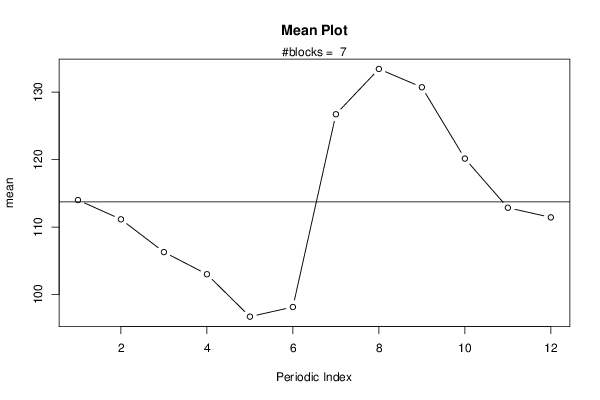PNG link Postscript link PDF link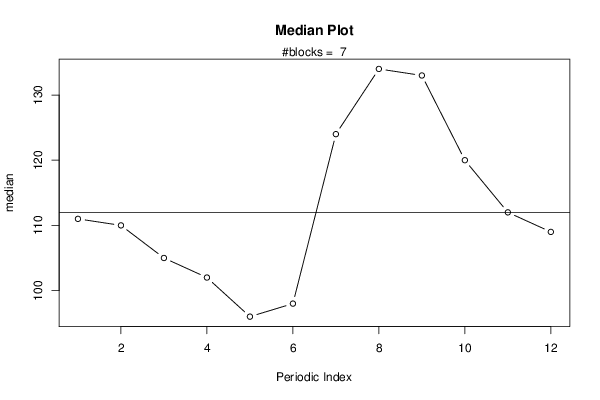PNG link Postscript link PDF link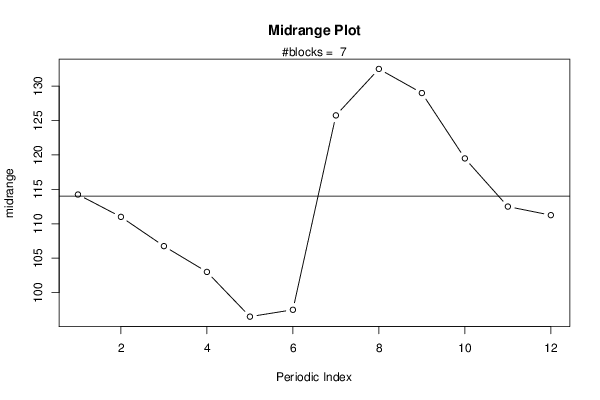PNG link Postscript link PDF link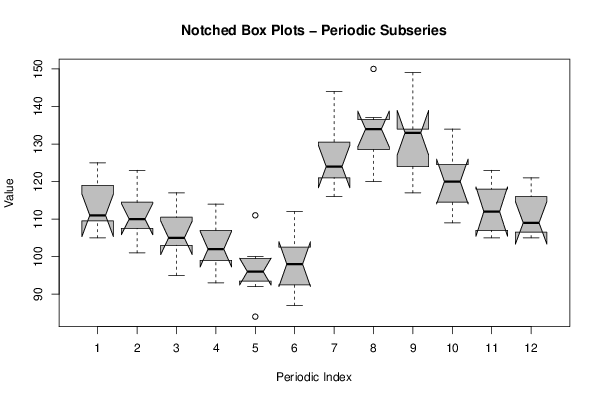PNG link Postscript link PDF link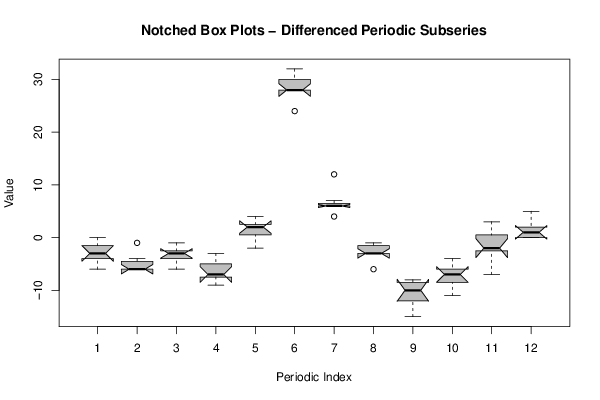PNG link Postscript link PDF link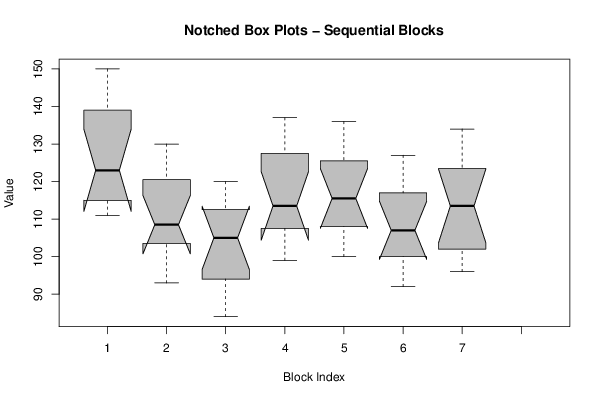PNG link Postscript link PDF link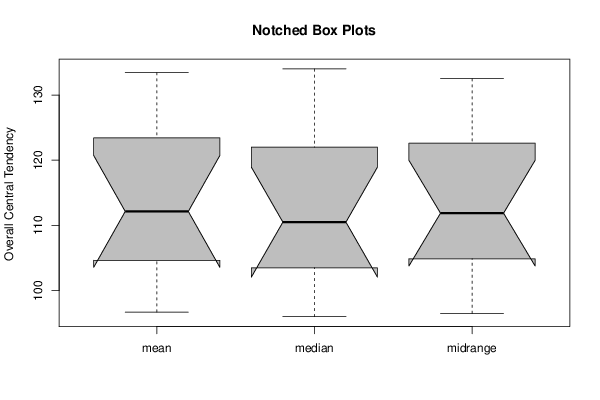PNG link Postscript link PDF link

Parameters (Session):
par1 = 12 ;
Parameters (R input):
par1 = 12 ;
R code (references can be found in the software module):
par1 <- as.numeric(par1)(n <- length(x))(np <- floor(n / par1))arr <- array(NA,dim=c(par1,np+1))darr <- array(NA,dim=c(par1,np+1))ari <- array(0,dim=par1)dx <- diff(x)j <- 0for (i in 1:n){j = j + 1ari[j] = ari[j] + 1arr[j,ari[j]] <- x[i]darr[j,ari[j]] <- dx[i]if (j == par1) j = 0}ariarrdarrarr.mean <- array(NA,dim=par1)arr.median <- array(NA,dim=par1)arr.midrange <- array(NA,dim=par1)for (j in 1:par1){arr.mean[j] <- mean(arr[j,],na.rm=TRUE)arr.median[j] <- median(arr[j,],na.rm=TRUE)arr.midrange[j] <- (quantile(arr[j,],0.75,na.rm=TRUE) + quantile(arr[j,],0.25,na.rm=TRUE)) / 2}overall.mean <- mean(x)overall.median <- median(x)overall.midrange <- (quantile(x,0.75) + quantile(x,0.25)) / 2bitmap(file='plot1.png')plot(arr.mean,type='b',ylab='mean',main='Mean Plot',xlab='Periodic Index')mtext(paste('#blocks = ',np))abline(overall.mean,0)dev.off()bitmap(file='plot2.png')plot(arr.median,type='b',ylab='median',main='Median Plot',xlab='Periodic Index')mtext(paste('#blocks = ',np))abline(overall.median,0)dev.off()bitmap(file='plot3.png')plot(arr.midrange,type='b',ylab='midrange',main='Midrange Plot',xlab='Periodic Index')mtext(paste('#blocks = ',np))abline(overall.midrange,0)dev.off()bitmap(file='plot4.png')z <- data.frame(t(arr))names(z) <- c(1:par1)(boxplot(z,notch=TRUE,col='grey',xlab='Periodic Index',ylab='Value',main='Notched Box Plots - Periodic Subseries'))dev.off()bitmap(file='plot4b.png')z <- data.frame(t(darr))names(z) <- c(1:par1)(boxplot(z,notch=TRUE,col='grey',xlab='Periodic Index',ylab='Value',main='Notched Box Plots - Differenced Periodic Subseries'))dev.off()bitmap(file='plot5.png')z <- data.frame(arr)names(z) <- c(1:np)(boxplot(z,notch=TRUE,col='grey',xlab='Block Index',ylab='Value',main='Notched Box Plots - Sequential Blocks'))dev.off()bitmap(file='plot6.png')z <- data.frame(cbind(arr.mean,arr.median,arr.midrange))names(z) <- list('mean','median','midrange')(boxplot(z,notch=TRUE,col='grey',ylab='Overall Central Tendency',main='Notched Box Plots'))dev.off()